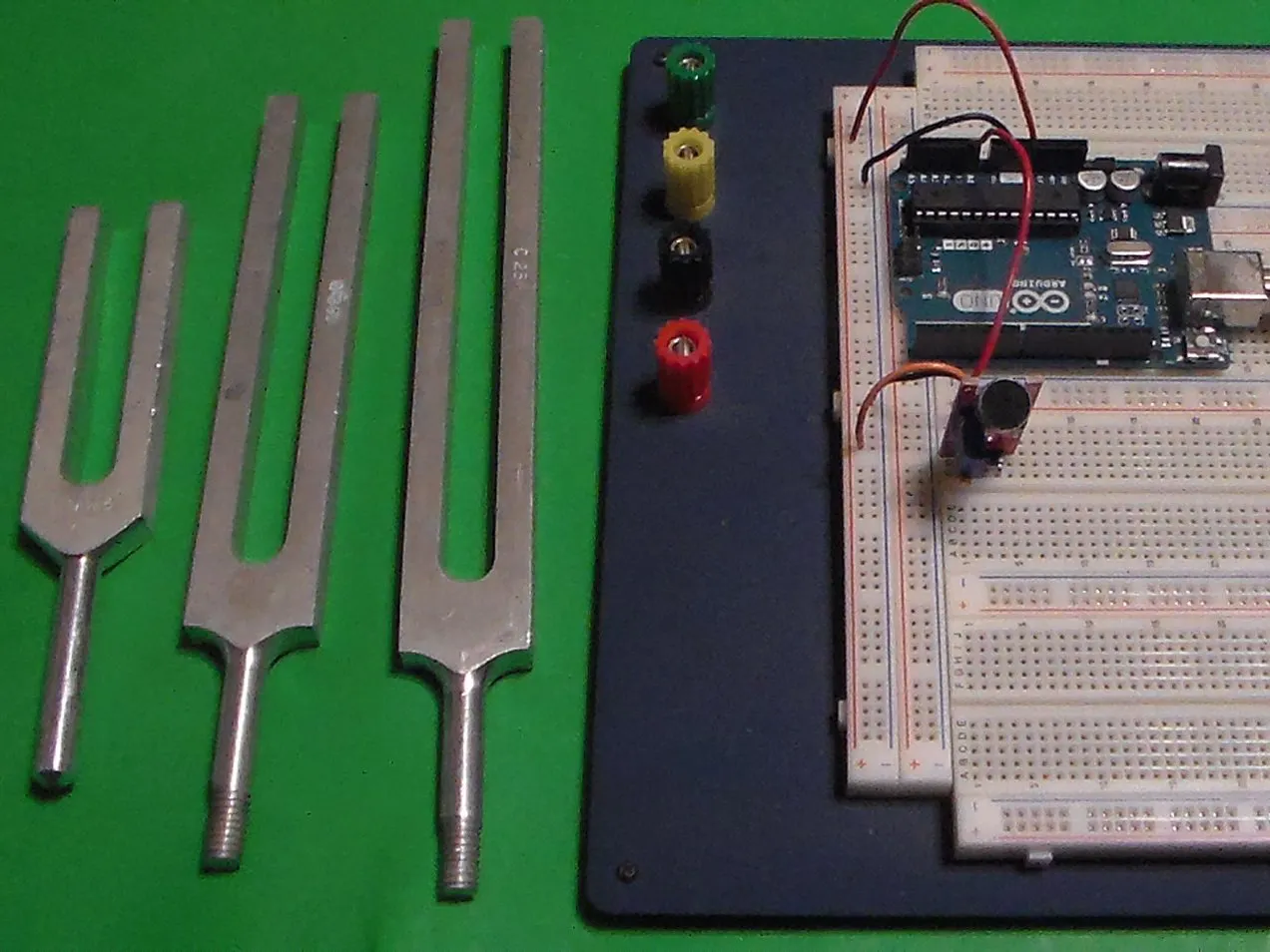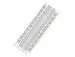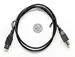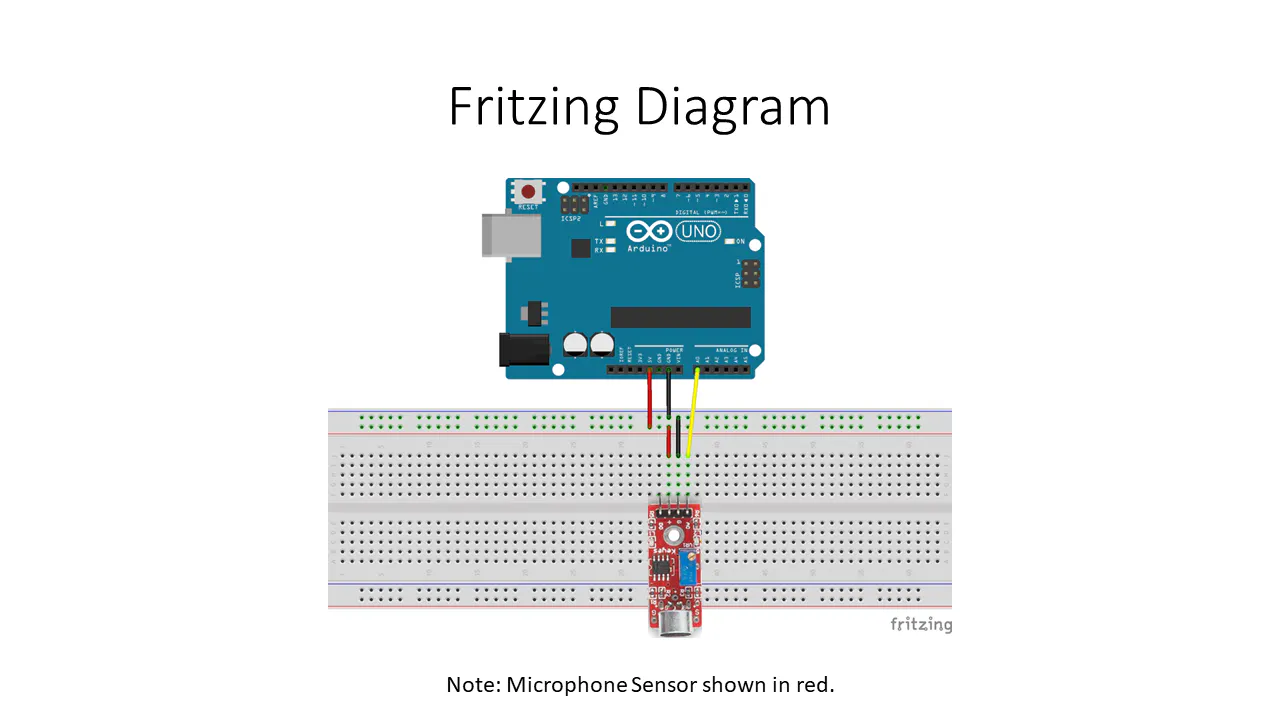# Audio Frequency Detector

This Arduino project displays the approximate frequency of the loudest sound detected by a sound detection module.

IntermediateFull instructions provided6,992## Things used in this project

### Hardware componentsArduino UNO & Genuino UNO
×1
 DEVMO High Sensitivity Sound Detection Module for Arduino
×1×1
 Computer
×1USB-A to B Cable
×1Jumper wires (generic)
×1

## Schematics

### Audio Frequency Detector

This Arduino project displays the approximate frequency of the loudest sound detected by a sound detection module.## Code

### AudioFrequencyDetectorV1-0.ino

Arduino
This code/sketch makes displays the approximate frequency of the loudest sound detected by a sound detection module.
```/*
File/Sketch Name: AudioFrequencyDetector

Version No.: v1.0 Created 12 December, 2019

Original Author: Clyde A. Lettsome, PhD, PE, MEM

Description:  This code/sketch makes displays the approximate frequency of the loudest sound detected by a sound detection module. For this project, the analog output from the
sound module detector sends the analog audio signal detected to A0 of the Arduino Uno. The analog signal is sampled and quantized (digitized). A Fast Fourier Transform (FFT) is
then performed on the digitized data. The FFT converts the digital data from the approximate discrete-time domain result. The maximum frequency of the approximate discrete-time
domain result is then determined and displayed via the Arduino IDE Serial Monitor.

Note: The arduinoFFT.h library needs to be added to the Arduino IDE before compiling and uploading this script/sketch to an Arduino.

License: This program is free software; you can redistribute it and/or modify it under the terms of the GNU General Public License (GPL) version 3, or any later

Notes: Copyright (c) 2019 by C. A. Lettsome Services, LLC

*/

#include "arduinoFFT.h"

#define SAMPLES 128             //SAMPLES-pt FFT. Must be a base 2 number. Max 128 for Arduino Uno.
#define SAMPLING_FREQUENCY 2048 //Ts = Based on Nyquist, must be 2 times the highest expected frequency.

arduinoFFT FFT = arduinoFFT();

unsigned int samplingPeriod;
unsigned long microSeconds;

double vReal[SAMPLES]; //create vector of size SAMPLES to hold real values
double vImag[SAMPLES]; //create vector of size SAMPLES to hold imaginary values

void setup()
{
Serial.begin(115200); //Baud rate for the Serial Monitor
samplingPeriod = round(1000000*(1.0/SAMPLING_FREQUENCY)); //Period in microseconds
}

void loop()
{
/*Sample SAMPLES times*/
for(int i=0; i<SAMPLES; i++)
{
microSeconds = micros();    //Returns the number of microseconds since the Arduino board began running the current script.

vReal[i] = analogRead(0); //Reads the value from analog pin 0 (A0), quantize it and save it as a real term.
vImag[i] = 0; //Makes imaginary term 0 always

/*remaining wait time between samples if necessary*/
while(micros() < (microSeconds + samplingPeriod))
{
//do nothing
}
}

/*Perform FFT on samples*/
FFT.Windowing(vReal, SAMPLES, FFT_WIN_TYP_HAMMING, FFT_FORWARD);
FFT.Compute(vReal, vImag, SAMPLES, FFT_FORWARD);
FFT.ComplexToMagnitude(vReal, vImag, SAMPLES);

/*Find peak frequency and print peak*/
double peak = FFT.MajorPeak(vReal, SAMPLES, SAMPLING_FREQUENCY);
Serial.println(peak);     //Print out the most dominant frequency.

/*Script stops here. Hardware reset required.*/
while (1); //do one time
}
```

## Credits

### lbf20012001

5 projects • 13 followers